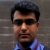# Using Probability Plot and Z Table

Six Sigma – iSixSigma Forums General Forums New to Lean Six Sigma Using Probability Plot and Z Table

Viewing 2 posts - 1 through 2 (of 2 total)
• Author
Posts
• #55008Steven Smith
Guest

Hi, am trying to answer this questions:

A random sample yielded the measurements 9.4, 10.6, 8.1, 12.2, 15.9, 9.0, 9.1, 10.0, 11.7, 11.0.

1. Determine the sample mean and the 95% confidence interval for the population mean.
2. Determine the sample standard deviation and the 95% confidence interval for the population standard deviation.
3.Display the data using a histogram and normal probability plots.
4. Estimate the response that is exceeded 10% of the time (using probability plot and Z table (Table A). Comment on the validity of the analysis and results.
5. Create a process capability/performance report for a specification of 10.0 ± 4.0. Comment on the validity of the analysis and results.

I’m very very stuck on number 4: “Estimate the response that is exceeded 10% of the time (using probability plot and Z table (Table A). Comment on the validity of the analysis and results.”

I’m not sure what it’s asking or how to tackle the problem. Can someone help please?

0
#198231Amit Kumar Ojha
Participant

For the first three points you just need to input the data in any of the statitical softwares and get the descriptive statistics. By default SPSS or Minitab gives the 95% confidence interval for mean and standard deviation.
Attaching the output for your data obtained through SPSS. You may further refer Statistics for Management by Levin and Rubin in order to learn calculations.

0
Viewing 2 posts - 1 through 2 (of 2 total)

You must be logged in to reply to this topic.